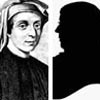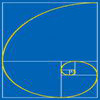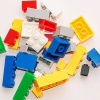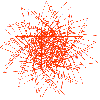# Resources tagged with: Fibonacci sequence

Filter by: Content type:
Age range:
Challenge level:

There are 30 NRICH Mathematical resources connected to Fibonacci sequence, you may find related items under Patterns, Sequences and Structure.

Broad Topics > Patterns, Sequences and Structure > Fibonacci sequence### 1 Step 2 Step

##### Age 11 to 14Challenge Level

Liam's house has a staircase with 12 steps. He can go down the steps one at a time or two at time. In how many different ways can Liam go down the 12 steps?### Fibs

##### Age 11 to 14Challenge Level

The well known Fibonacci sequence is 1 ,1, 2, 3, 5, 8, 13, 21.... How many Fibonacci sequences can you find containing the number 196 as one of the terms?### Fibonacci Surprises

##### Age 11 to 14Challenge Level

Play around with the Fibonacci sequence and discover some surprising results!### Farey Fibonacci

##### Age 16 to 18 ShortChallenge Level

Investigate Farey sequences of ratios of Fibonacci numbers.### Building Gnomons

##### Age 14 to 16Challenge Level

Build gnomons that are related to the Fibonacci sequence and try to explain why this is possible.### Simple Train Journeys

##### Age 5 to 11Challenge Level

How many different journeys could you make if you were going to visit four stations in this network? How about if there were five stations? Can you predict the number of journeys for seven stations?### First Forward Into Logo 11: Sequences

##### Age 11 to 18Challenge Level

This part introduces the use of Logo for number work. Learn how to use Logo to generate sequences of numbers.### Whirling Fibonacci Squares

##### Age 11 to 16

Draw whirling squares and see how Fibonacci sequences and golden rectangles are connected.### Colour Building

##### Age 11 to 14Challenge Level

Using only the red and white rods, how many different ways are there to make up the other rods?### Plus or Minus

##### Age 16 to 18Challenge Level

Make and prove a conjecture about the value of the product of the Fibonacci numbers $F_{n+1}F_{n-1}$.### Pythagorean Fibs

##### Age 16 to 18Challenge Level

What have Fibonacci numbers got to do with Pythagorean triples?### Fibonacci Fashion

##### Age 16 to 18Challenge Level

What have Fibonacci numbers to do with solutions of the quadratic equation x^2 - x - 1 = 0 ?### Golden Mathematics

##### Age 16 to 18

A voyage of discovery through a sequence of challenges exploring properties of the Golden Ratio and Fibonacci numbers.### The Golden Ratio, Fibonacci Numbers and Continued Fractions.

##### Age 14 to 16

An iterative method for finding the value of the Golden Ratio with explanations of how this involves the ratios of Fibonacci numbers and continued fractions.### Golden Fractions

##### Age 16 to 18Challenge Level

Find the link between a sequence of continued fractions and the ratio of succesive Fibonacci numbers.### Sheep Talk

##### Age 7 to 11Challenge Level

In sheep talk the only letters used are B and A. A sequence of words is formed by following certain rules. What do you notice when you count the letters in each word?### Last Biscuit

##### Age 11 to 18Challenge Level

Can you find a strategy that ensures you get to take the last biscuit in this game?### Leonardo of Pisa and the Golden Rectangle

##### Age 7 to 16

Leonardo who?! Well, Leonardo is better known as Fibonacci and this article will tell you some of fascinating things about his famous sequence.### Fibonacci's Three Wishes 2

##### Age 7 to 14

Second of two articles about Fibonacci, written for students.### Golden Fibs

##### Age 16 to 18Challenge Level

When is a Fibonacci sequence also a geometric sequence? When the ratio of successive terms is the golden ratio!### Fibonacci Factors

##### Age 16 to 18Challenge Level

For which values of n is the Fibonacci number fn even? Which Fibonnaci numbers are divisible by 3?### Ordered Sums

##### Age 14 to 16Challenge Level

Let a(n) be the number of ways of expressing the integer n as an ordered sum of 1's and 2's. Let b(n) be the number of ways of expressing n as an ordered sum of integers greater than 1. (i) Calculate. . . .### Stringing it Out

##### Age 14 to 16Challenge Level

Explore the transformations and comment on what you find.##### Age 11 to 16Challenge Level

Using logo to investigate spirals### LOGO Challenge - Circles as Bugs

##### Age 11 to 16Challenge Level

Here are some circle bugs to try to replicate with some elegant programming, plus some sequences generated elegantly in LOGO.### Continued Fractions I

##### Age 14 to 18

An article introducing continued fractions with some simple puzzles for the reader.### Gnomon Dimensions

##### Age 14 to 16Challenge Level

These gnomons appear to have more than a passing connection with the Fibonacci sequence. This problem ask you to investigate some of these connections.### Paving Paths

##### Age 11 to 14Challenge Level

How many different ways can I lay 10 paving slabs, each 2 foot by 1 foot, to make a path 2 foot wide and 10 foot long from my back door into my garden, without cutting any of the paving slabs?### Golden Powers

##### Age 16 to 18Challenge Level

You add 1 to the golden ratio to get its square. How do you find higher powers?### Room Doubling

##### Age 7 to 11Challenge Level

Investigate the different ways you could split up these rooms so that you have double the number.